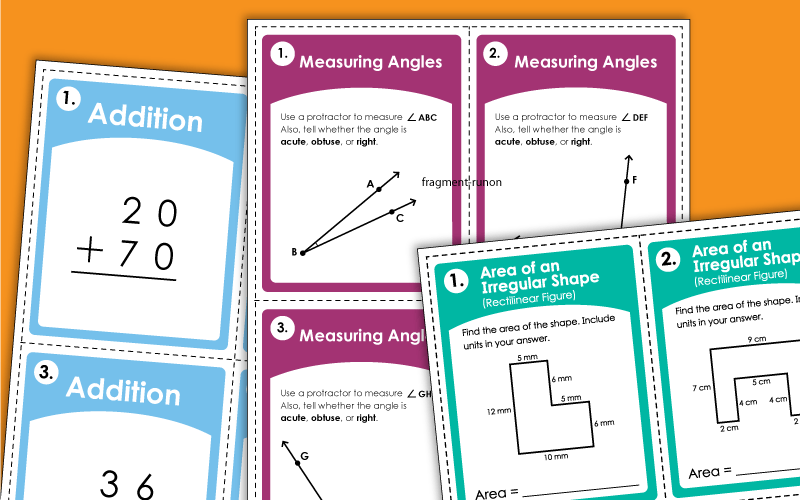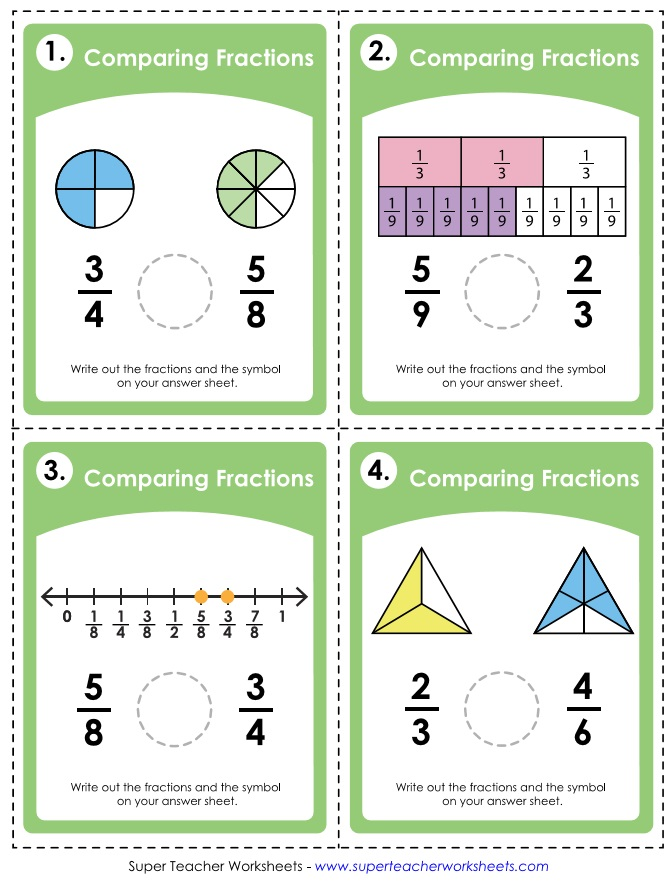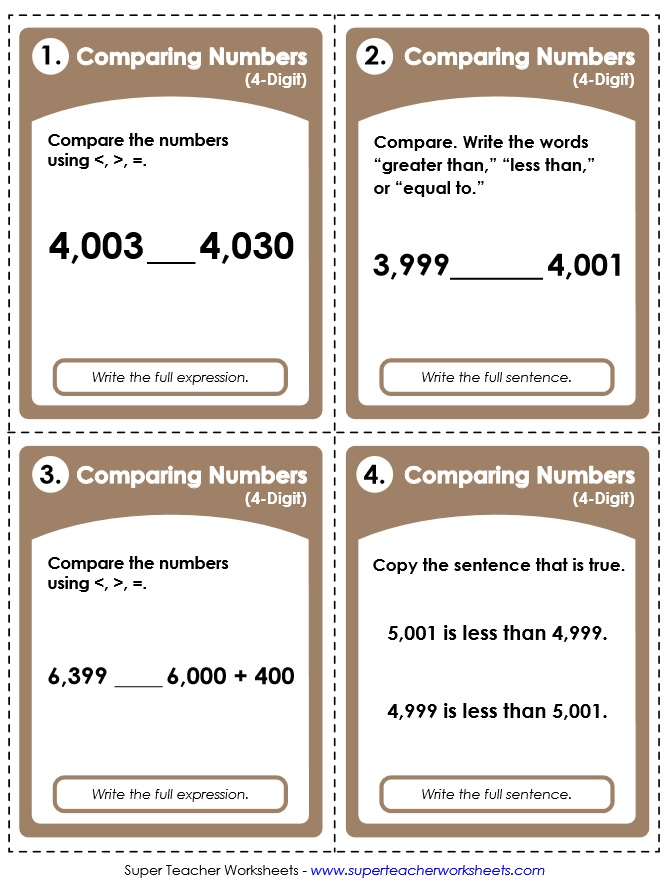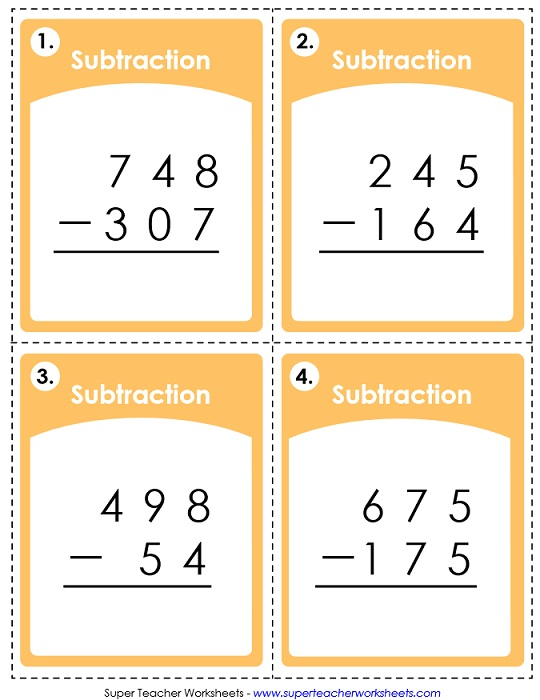There are countless ways to use the task cards below. You can create classroom learning centers. Post them as daily or weekly challenges. Use them with your whiteboard's document camera. Laminate them and let students write on them with dry-erase markers. The possibilities are endless.This file has 30 task cards for teaching absolute value of integers.
This task card set has 30 addition problems. Students do not regroup (carry) in these problems.
These two-digit addition problems will require students to regroup (carry ones).
On these cards, students will solve addition problems with addends up to 3 digits.
This set of 30 cards can be used to review addition with 5-digit addends. Problems are vertical.
Look carefully at the base-10 block models and the partial hundreds charts to figure out what is "10 more."
Add a multiple of 10 to each 2-digit number. Each card has PV block models or a hundreds chart.
Measure each angle with a protractor. Write the measurement of each and tell if it is acute, obtuse, or right.
Identify whether angles are acute, right, or obtuse using these printable task cards.
This version of the "counting square units" task cards is slightly harder than the version listed above.
Given the radius or diameter, calculate the areas of the circles.
Practice finding the area of rectangles by multiplying the length by the width.
In this deck you'll find a collection of 30 "area of a trapezoid" problems for students to solve.
Find the area of the irregular shape (rectilinear figure) by finding the area of each rectangle within the shape and adding those areas together.
This set contains 30 task cards. Find the areas of each rectangle within the shape by multiplying decimals. Then add them together to find the area of the irregular shape (rectilinear figure).
This math task card set has 30 "greater than / less than / equal to" problems for kids to solve.
this task card set will help students practice comparing 3-digit numbers using the symbols for "greater than," less than," and "equal to."
Each of the cards in this set has 4-digit numbers for students to compare.
Use these task cards for learning centers or class games. Includes 30 "greater/less/equal" problems with five-digit numbers.
These task cards can be used to help students practice using the greater than, less than, and equal to symbols to compare numbers with six digits.
Write a contraction for the underlined words.
Help students practice converting inches, feet, and yards with these task cards.
Help students practice converting between centimeters, millimeters, and meters with this set of task cards.
This set of 30 task cards features a variety of conversion problems, including simple conversions, comparing measurements of different units, and word problems.
Use this set of task cards to help students practice converting pounds and ounces.
Use these task cards with students to help them practice converting between pounds, ounces, and tons.
Identify, name decimals, and write numbers in decimal notation. Includes tenths and hundredths.
These cards have 30 basic division problems, with remainders. (example: 8 divided by 38)
On these PDF task cards, students will divide numbers with 3-digit dividends. (example: 127 divided by 2)
With these task cards, students will practice dividing using 2-digit divisors. The problems are written traditionally, horizontally, and as fractions.
Solve long division problems with 3-digit dividends and 2-digit divisors. All problems have remainders.
With the problems on this PDF, you can try your hand at division of mixed numbers.
Divide decimals by 10, 100, and 1,000 with these printable task cards.
Learn about exponents with this set. Write each repeated multiplication fact as an exponent. Then compare exponents. Then write exponents in standard form.
Students will determine which basic fractions are shown by the illustrations on the cards.
Compare pairs of fractions. Use the illustration models to help.
Compare pairs of fractions using the mathematical symbols <, >, and =.
This is a set of 30 task cards on simplifying (reducing) fractions.
Add fractions with unlike denominators and write the sum on the answer page.
Calculate the fraction of a set. For example: What is 5/6 of 48? This set has 30 cards, which includes 15 word problems.
Switch improper fractions to mixed numbers, and mixed numbers to improper fractions.
Choose the correct work (you're or your) to complete the sentences shown.
A set of 30 task cards to practice subtracting mixed numbers with unlike denominators.
On these task cards, students will find the values of the coins shown. Each card shows only a few, easy-to-count coins. Example: A dime and a penny = \$0.11
Add up the values of the coins to find the total. (example: 3 quarters + 2 dimes + 3 pennies = \$0.98)
Your students will use these cards alone, in small groups, or as a class and count the bills and coins to find the amount. Example: 2 one dollar bills + 2 quarters + 2 pennies = \$2.28.
Round money amounts up to the next dollar. Example: To buy a sweater that costs \$8.19, you would need \$9.00.
For these activities, students must find the missing factor for each basic multiplication fact.
Students write the basic multiplication fact that corresponds with the arrays shown. (Basic facts 0s through 9s.)
Multiply double-digit numbers by single-digit numbers on these 30 task cards.
Multiply three-digit by 1-digit numbers on these printable task cards.
With these printable task cards students can find products of pairs of 2-digit numbers.
With these cards, students can try to multiply pairs of three-digit numbers.
Practice multiplying by 6s with these handy task cards.
Use these task cards to help students practice multiplying by 7s.
Use these task cards to review multiplying by 8s with your students.
Review multiplying by 9s with this set of task cards.
Review multiplying by 10s using these task cards.
Practice multiplying decimals to the tenths and hundredths place by single digit numbers with the help of these task cards.
With these printable task cards, students will practice multiplying two digit numbers by decimals to the tenths and hundredths.
On these cards, students will practice multiplying pairs of fractions.
With these task cards, your students can review multiplication of mixed numbers.
These problems cards have money amounts as the first factor, and a single-digit number for the second factor. (example: \$4.56x3)
Read the sentences on the cards. Each one has exactly one noun in it. Identify the nouns.
Choose the best collective noun to complete each sentence.
Here are discussion cards that can be used for discussion of any short story, novel, or chapter book.
Here are 30 cards with 2-digit numbers. Students round each number to the nearest ten.
(example: Round 37 to the nearest ten.)
Round each 2 or 3-digit number to the nearest ten. (example: Round 148 to the nearest ten.)
Round each number to the nearest hundred. (example: Round 329 to the nearest hundred.)
Round each 3 or 4-digit number number to the nearest hundred. (example: Round 2,310 to the nearest hundred.)
These have a mixture of 2 3, and 4-digit numbers. Round to the nearest ten or hundred, as per the instructions.
Round each of these numbers to the nearest tens, hundreds, or thousands place.
Round each number to the nearest tenth using these printable task cards
Round the each number to the nearest whole number, tenth, or hundredth.
With these cards, students will practice subtracting two-digit numbers. These problems do not require students to regroup of borrow.
Subtract pairs of 2-digit numbers. Most of these problems require students to regroup (borrow).
Subtract pairs of three-digit numbers on these student task cards. Most problems require students to borrow/regroup/rename.
With these 30 task cards, students can practice subtracting 4-digit numbers.
Here you'll find 4-digit subtraction problems. All require students to borrow across the number zero.
Subtract 10 from 2-digit numbers using the place value block models and the partial hundreds charts.
(example: 35-10)
Subtract multiples of ten from 2-digit numbers using a hundreds chart or base-10 blocks.
(examples: 87-40=47 and 92-50=42)
This set has 12 cards with clocks on them. The clocks show "top of the hour" times. (examples: 3:00, 7:00, 12:00)
This one has 24 different clocks. Each one shows a time in half-hour intervals. (examples: 5:30, 12:00, 1:30, 9:00)
These clocks have times with 5 minute intervals. (example: 8:50, 10:25, 1:05) There are thirty clocks in this set.
On these cards, you'll see clocks with one-minute intervals.(examples: 1:34, 12:57, 9:02) Students must read the clock and tell what time is shown.
Determine how many cubes are in each shape to estimate the volume.
Calculate the volumes of the given cylinders. Includes 15 cards with picture models and 15 phrase problems.
Multiply length times width times height to find the volumes of the rectangular prisms.
This is an advanced-level worksheets for calculating volumes of rectangular prisms. Unlike the basic level, each shape has one or more decimal measurements.

Scoot! Games

This page has Scoot! games. This is a whole-class card game for practicing math concepts.

"I have / Who has" Games

"I have... Who has..." is a whole-class card game in which students answer math facts quickly without breaking the chain.

Classroom Scavenger Hunts

For this game, students search the classroom for hidden facts or math problems to solve.

## Images of Our PrintablesMy Account
Site Information